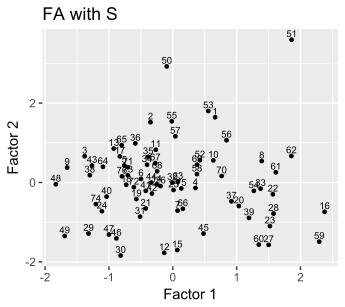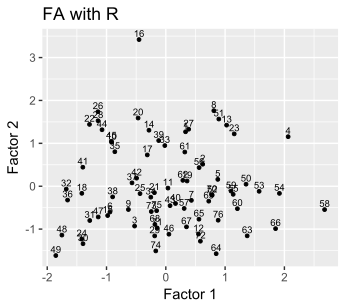# Q1: 9.12

$$\mathbf{S_n} = \frac{23}{24} \mathbf{S} = \begin{pmatrix} 0.01061 & 0.00768 & 0.00782 \\ 0.00768 & 0.00615 & 0.00575 \\ 0.00782 & 0.00575 & 0.00649 \\ \end{pmatrix}$$

## (a) Specific Variances

$$\mathbf{S_n} \approx \hat{\mathbf{L}} \hat{\mathbf{L}}^T + \hat{\mathbf{\Psi}}$$, where $$diag(\mathbf{S_n}) = diag(\hat{\mathbf{L}} \hat{\mathbf{L}}^T) + diag(\hat{\mathbf{\Psi}})$$

Hence, $$\hat{\mathbf{\Psi}} = diag(\hat{\mathbf{\Psi}}) = diag(\mathbf{S_n} - \hat{\mathbf{L}} \hat{\mathbf{L}}^T)$$

$$\hat{\mathbf{\Psi}} = \begin{pmatrix} 0.000166 & 0.000000 & 0.000000 \\ 0.000000 & 0.000495 & 0.000000 \\ 0.000000 & 0.000000 & 0.000639 \\ \end{pmatrix}$$

## (b) Communalities

$$\sigma_{ii} = \ell_{i1}^2 + \ell_{i2}^2 + ~...~ + \ell_{im}^2 + \psi_i$$

$$h_i^2 = \ell_{i1}^2 + \ell_{i2}^2 + ~...~ + \ell_{im}^2 = \ell_{i1}^2$$

$$h_1^2 = 0.0104, ~ h_2^2 = 0.0057, ~ h_3^2 = 0.0059$$

## (c) Proportion of variance explained by the factor

$$\frac{s_{11} + s_{22} + ~...~ + s_{pp}}{h_i^2} = 0.9441$$

## (d) Residual Matrix

$$\mathbf{S_n} - \mathbf{\hat{L}}\mathbf{\hat{L}}^T - \mathbf{\hat{\Psi}} = \begin{pmatrix} 0.000000 & -0.000166 & -0.000164 \\ -0.000495 & 0.000000 & -0.000493 \\ -0.000637 & -0.000637 & 0.000000 \\ \end{pmatrix}$$

# Q2: 9.32

## (a) S PC

RC1 RC2 RC3
YrHgt 0.566 0.734 0.87
FtFrBody 31.179 83.935 24.022
PrctFFB 0.601 1.488 2.742
Frame 0.296 0.39 0.415
BkFat 0.014 -0.006 -0.055
SaleHt 1.021 0.934 0.82
SaleWt 122.551 39.068 -17.48

## (b) S ML

ML1 ML2 ML3
YrHgt -0.001 0.581 1.629
FtFrBody 21.802 84.458 31.383
PrctFFB -0.253 2.155 1.056
Frame 0.019 0.307 0.817
BkFat 0.033 -0.015 -0.027
SaleHt 0.498 0.841 1.532
SaleWt 119.136 33.925 38.8

## (c) R PC

RC1 RC3 RC2
YrHgt 0.941 0.27 -0.082
FtFrBody 0.447 0.794 0.205
PrctFFB 0.262 0.859 -0.295
Frame 0.938 0.219 -0.028
BkFat -0.231 -0.339 0.812
SaleHt 0.833 0.419 0.109
SaleWt 0.352 0.43 0.722

## (d) R ML

ML1 ML2 ML3
YrHgt 0.941 0.286 0.164
FtFrBody 0.414 0.505 0.553
PrctFFB 0.231 0.947 0.212
Frame 0.891 0.251 0.18
BkFat -0.256 -0.514 0.273
SaleHt 0.755 0.269 0.434
SaleWt 0.253 -0.05 0.879

## (e) Compare S & R

The results obtained using covariance matrices are hard to interpret here. Since there are three kinds of units used: pound, inch, and self-defined scales(1-8), the factor loadings on some variables are very large and others small.

Interpretation of the factors is straightforward using results obtained from the covariance matrix.
By (c) and (d), 1. factor 1 has larger loadings on YrHgt, Frame, and SaleHt, which are all related to the heights of the bulls. 2. Factor 2 has large loadings on FtFrBody and PrctFFB and is negatively related to BkFat in both methods(PC & ML) of factor analysis. It might be called a lean factor. 3. In both PC & ML method of factor analysis, the loadings of factor 3 is large on SaleWt. The loading is also large on BkFat from the PC method, and the loading is medium on FtFrBody from the ML method. Factor 3 might be related to the weights of the bulls.

## (f) scatter plots of factor2 vs factor1 in (a) and (c)

The point, 51, in the scatter plot on the left and the point, 16, in the scatter plot on the right seem to be outliers.